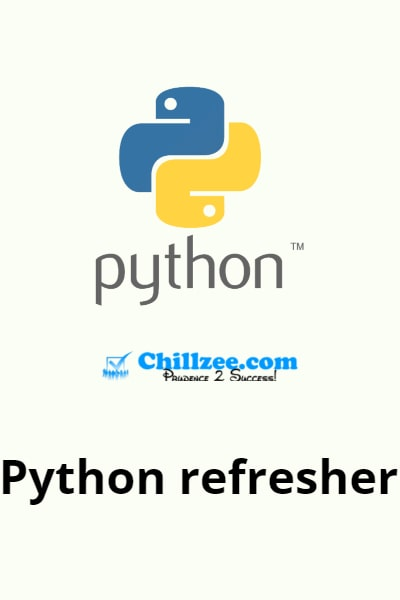# Python Refresher - Numbers (1 minute)

## Numbers

• Mathematical operations like addition (+), subtraction (-), multiplication (*), division (/) and exponential (**) can be performed on number in python.

• Python supports operator hierarchy i.e. for example, multiplication is performed before addition and so on.

Example:

3+3+3*4

Returns 18 because 3*4 is performed first and then the addition is performed.

• Python ignores white spaces between numbers and operators.

Example:

3   + 3 +3  *4

Returns 18.

• Even when a division returns a whole number, Python returns it as a float.

Example:

6/2

Returns 3.0 and not 3.

• If a mathematic operation is performed using a float number, Python always returns the output as a float number, even if the output is a whole number.

Example:

3*5

Returns 15.

While,

3.2*5

Returns 16.0 and not 16.Author: Oxygen
Other articles by this author

### Chillzee Tag Cloud

Your browser doesn't support this tag cloud